Classes FollowEduncle posted an Article
April 19, 2018 • 12:00 am

# CBSE Class 10 Maths Syllabus 2018 & Tips to Attempt Paper!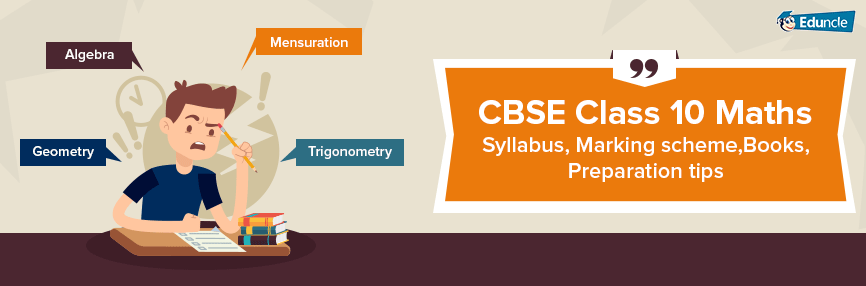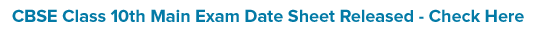Hello Aspirants!!!

Want to maximize your score in the CBSE Class 10th Board Exam? Being a student of Class 10th, it is high time that you should give a shape to your preparation as CBSE Class 10th Board exam are around the corner. The Class 10th Maths under the CBSE board is the easy one though many students face difficulty in scoring good marks in this.

Previously, the board exams were not mandatory for the Class 10. But it has been reintroduced from the session 2017-18. So, it is very important to be updated with the latest syllabus and exam pattern. Also, students need to study the Mathematics subject with a proper study plan.

So, to score well in the CBSE Class 10th Board Exam you need to be aware of the latest happenings for the exam.

Hence, here in this blog, we are going to discuss every small and important details that will help you in preparing for the CBSE Class 10 Maths.

CBSE Class 10 Result Date 2018 and Exam Schedule

CBSE Class 10th Syllabus (All Subjects)

## CBSE Syllabus for Class 10 Maths

The exam syllabus is important as it will help students in preparing for the exam according to the weightage of the topics. In this article, you will get the complete syllabus of the CBSE Class 10 Maths.

The CBSE has introduced two levels of Mathematics exam for CBSE Class 10th from the Academic Session ending March, 2020 onwards.

This year as per the CBSE’s new curriculum, CBSE Class 10 Board exams are obligatory. The new assessment structure will have 80 marks for the annual examination along with the 20 marks of Internal Assessment (IA).

The detailed CBSE Class 10 Maths syllabus is as follows:

### Unit I: Number Systems

Real Numbers

Euclid’s division lemma; The fundamental theorem of arithmetic; Terminating/Non-terminating recurring decimals.

### Unit II: Algebra

Polynomials

Zeroes and coefficients of the quadratic polynomials, Division algorithm for polynomial

Pair of Linear Equations in Two Variables

Algebraic method of solving the pair of linear equation-by substitution, by elimination & by cross multiplication method; Equations reducible to linear equations.

Standard form of quadratic equation; Solutions of quadratic equations by factorization, by completing the square; Nature of roots

Arithmetic Progressions

Introduction; Derivation of the nth term and sum of the first n terms of an A.P.

### Unit III: Coordinate Geometry

Lines

Graph of linear equations; Distance Formula, Section formula; Area of the triangle.

### Unit IV: Geometry

Triangles

Similar figures; Similar triangles-(Prove) If a line is drawn parallel to one side of a triangle, the other two sides are divided in the same ratio; If a line divides two sides of a triangle in the same ratio, the line is parallel to the third side; Basic proportionality theorem; Criteria of similarity; Area of similar triangles; Pythagoras theorem and its converse.

Circles

Tangent to a circle and its construction; Number of tangent from a point to a circle;

Constructions

Division of line segment; Construction of similar triangle; Constructions of tangents to a circle.

### Unit V: Trigonometry

Introduction to trigonometry

Trigonometric ratios of an acute angle; Values of the trigonometric ratios of 30°, 45°, and 60°. The relationship between ratios.

Trigonometric identities

Trigonometric ratios of complementary angles; Proof and applications of the identities.

Heights and Distances

The angle of elevation; Angle of depression; Problems based on angles including only 30°, 45°, 60°.

### Unit VI: Mensuration

Areas related to Circles

Motivate the area of a circle; area of sectors and segments of a circle. Problems based on the areas and perimeter of the plane figures.

Surface Areas and Volumes

Surface areas and volumes of combinations of any two of the figures: cubes, cuboids, spheres, hemispheres and right circular cylinder/cones. Frustum of a cone; Conversion of one solid shape into another.

### Unit VII: Statistics and Probability

Statistics

Mean, median and mode of grouped data; Cumulative frequency graph.

Probability

Classical definition of probability; Simple problems on single events.

## CBSE Class 10 Maths Unit-Wise Weightage

The unit-wise weightage for the annual examination which is of the 80 marks is as follows:

 Units Unit Name Marks I Number Systems 06 II Algebra 20 III Coordinate Geometry 06 IV Geometry 15 V Trigonometry 12 VI Mensuration 10 VII Statistics & Probability 11 Total 80

The internal assessment which is of the 20 marks is divided into the three parts as follows:

 Internal Assessment 20 marks Periodic Test 10 marks Notebook Submission 05 marks Lab Practical 05 marks

### Question Paper Design for CBSE 10 Maths

The CBSE made it clear regarding the exam pattern along with the question paper design for the Board exam 2018. The following table mentions the question structure for the CBSE Class 10 Maths Exam:

 Section Number of Questions Marks per question A 6 1 B 6 2 C 10 3 D 8 4

## CBSE Class 10 Maths Books

The CBSE Syllabus for Class 10 Maths has a vital role in the preparation. Along with syllabus, study material and books play an important role in the preparation and gives you the latest and good number of practice questions. The list of the CBSE books for the Class 10 Maths is as follows:

Mathematics-Textbook for Class X-NCERT Publication

Guidelines for Mathematics Laboratory in Schools, Class X-CBSE Publication

Laboratory Manual- Mathematics, secondary stage-NCERT Publication

Mathematics exemplar problems for class X, NCERT publication

Mathematics for class X- R.D. Sharma

## CBSE Class 10 Maths Preparation tips

Students believe that to score well in the Maths is one of the toughest tasks, but it is not that hard to score if you practice regularly and keep a check on some essential tips and tricks. It is high time to focus on the preparation plan. Here we are going to discuss some of the preparation tips, which will help you to in achieving your goals.

### Don’t mug up the mathematics-

Learning the questions and trying to use them in the exam is one of the common mistakes by the students. Try to understand and practice the questions based on every topic of CBSE Class 10 Maths Text Book.

### Study according to a proper timetable-

With exam being around the corner, you need to be punctual not in your studies but in other leisure activities also. Follow a proper timetable for every day. Try to finish your CBSE Syllabus for Class 10 on time to boost your confidence.

### Understand the role of syllabus and exam pattern-

Having a clear understanding of the syllabus and exam pattern will give you the idea of the time you need to dedicate to each unit.

### Make Short notes for important formulas and theorems-

Every chapter of Maths consists of important formulas and theorems which are hard to revise at the time of the exam. So, create separate notes of important Maths formulas and theorems for Class 10th CBSE so that you can revise them easily at the time of the exam.

### Don’t ignore the figures and graphs-

However, Graphs and figures are not a major part of the exam, but they can help you to score more marks if you concentrate on them.

### Solve the CBSE practice papers and previous year papers-

Solving previous years papers and practice papers help you in practising the things in a limited time.

Solving the test papers will let you know your weak areas so that you can work on them. The more you practice, the more you become proficient in it.

### Try to solve the questions yourself-

Give your best while solving the questions, don’t see the solutions immediately after one attempt. Try at least 2-3 times to solve questions.

### Be consistent-

Always be consistent in your studies and focus on the other subjects along with the Maths, as these subjects have the same weightage as of the maths.

### Stay confident and be positive-

Always be confident about your approach and don’t lose hope even if you are not being able to score well in the practice exams.

## Paper Attempting Tips for CBSE Class 10 Maths

Now after the preparation for the maths exam, there are many things which a student need to keep in mind while attempting the question paper of the Class 10 Maths. Here we bring you some golden tips such as:

### Utilize the first 15 minutes very effectively-

The first 15 minutes are for reading the question paper. So, try to read all the questions and categorize the questions as easy, moderate & tough in your mind.

### Keep question paper and answer sheet clean and tidy-

To have a good impact on the examiner, you need to answer in a very clear and concise manner. Avoid cutting and overwriting in the answer sheet. Draw proper margins for rough work.

### Keep a close eye on the time-

Don’t waste much time on any question and keep track on the time and manage the time and questions left. However, don’t pressurize yourself for the time management as the question paper is not much longer.

### Weightage of the questions is important-

The time allocation during the exam should be based on the weightage of the questions. Try to attempt the 4 marks questions first.

In long questions, give special attention to the stepwise process of answering the questions. As described by the CBSE, there are marks for the step-wise answer. So, even if you are not able to answer correctly, writing the known steps will help you to score the little bit.

### Re-check the answers in the end-

Try to finish the question paper 10 minutes before the time and check the answers again for any silly mistakes.

We know that it is quite hard to remember all these points but try to improvise in the practice exams and Pre-Boards to see the changes yourself.

So, here we are done with the whole information regarding the CBSE Class 10 maths. We hope that we have covered all the important aspect regarding the Class 10 maths. If you have any doubt regarding this blog, you can ask your query in the comment section below.

All the best!!!!

You Might Also Like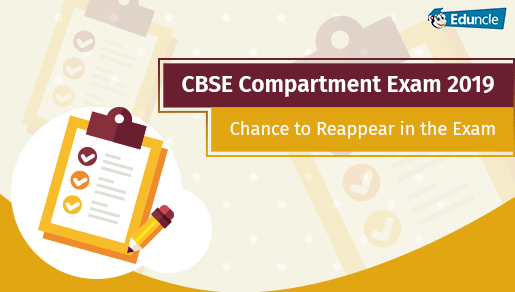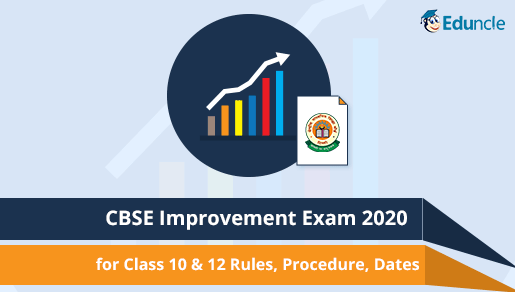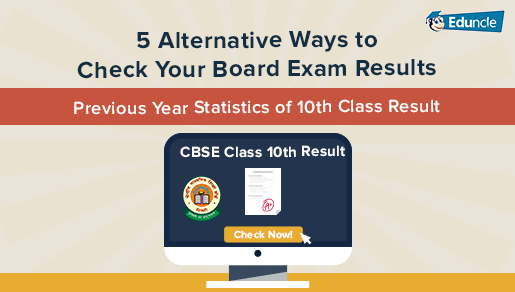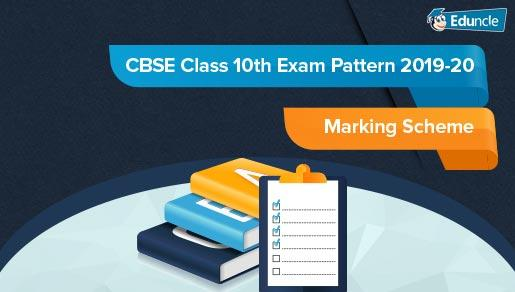Showing 2 comments out of 2
• Subhasmita Swain
Wow very nice I liked it so much Thanks for help...
Eduncle
Hello Subhasmita, Greetings from Eduncle! Thanks for the kind words and appreciation! We value your generous feedback. Kind Regards, Eduncle Team